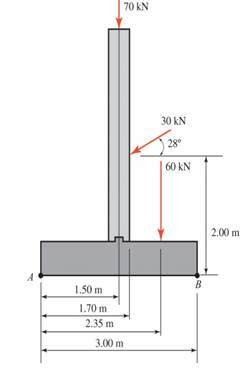### Create an Account

Home / Questions / For the concrete wall and footing shown a Calculate the algebraic summation of the moment...

# For the concrete wall and footing shown a Calculate the algebraic summation of the moments of the forces shown about point

For the concrete wall and footing shown:

a. Calculate the algebraic summation of the moments of the forces shown about point A.

b. Calculate the algebraic summation of the moments of the forces about point B.Jun 26 2020 View more View LessSubscribe To Get Solution